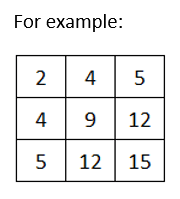# Input numbers in 2d array and check symmetric matrix or not in C

###### Two Dimensional Array - Question 10

In this question, we will see how to input numbers in a 3X3 integer matrix (2d array) and check whether it is a symmetric matrix or not in C programming. To know more about two dimensional array click on the two dimensional array lesson.

Q10) Write a program in C to input numbers in a 3X3 integer matrix (2d array) and check whether it is a symmetric matrix or not. Symmetric matrix is such a matrix whose row elements are exactly same as column elements.#### Program

``````#include <stdio.h>
#include <conio.h>

int main()
{
int a, r,c,x=0;

printf("Enter 9 numbers\n");
for(r=0; r<3; r++)
{
for(c=0; c<3; c++)
{
scanf("%d",&a[r][c]);
}
}

for(r=0; r<3; r++)
{
for(c=0; c<3; c++)
{
printf("%d ",a[r][c]);
if(a[r][c]==a[c][r])
{
x++;
}
}
printf("\n");
}

if(x==9)
{
printf("Symmetric Matrix");
}
else
{
printf("Not Symmetric Matrix");
}
return 0;
}``````

#### Output

```Enter 9 numbers
2
4
5
4
9
12
5
12
15
2 4 5
4 9 12
5 12 15
Symmetric Matrix```